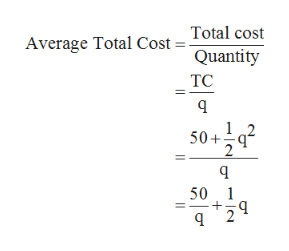# Suppose that each firm in a competitive industry has thefollowing costs:Total cost: TC=50 + 1/2q^2Marginal cost: MC=qwhere q is an individual firm’s quantity produced. The marketdemand curve for this product isDemand: QD = 120 – Pwhere P is the price and Q is the total quantity of the good.Currently, there are 9 firms in the market.a. What is each firm’s fixed cost? What is its variable cost?Give the equation for average total cost.b. Graph average total cost curve and the marginal cost curvefor q from 5 to 15. At what quantity is average total cost curve atits minimum? What us marginal cost and average total cost at thisquantity?c. Give the equation each firm’s supply curve.d. Give the equation for the market supply curve for the shortrun in which the number of firms is fixed.e. What is the equilibrium price and quantity for this market inthe short run?f. In this equilibrium, how much does each firm produce?Calculate each firm’s profit or loss. Is there incentive for firmsto enter or exit?g. In the long run with free entry and exit, what is theequilibrium price and quantity in this market?h. In this long run equilibrium, how much does each firmproduce? How many firms are in the market?

Question
23 views

Suppose that each firm in a competitive industry has thefollowing costs:

Total cost: TC=50 + 1/2q^2

Marginal cost: MC=q

where q is an individual firm’s quantity produced. The marketdemand curve for this product is

Demand: QD = 120 – P

where P is the price and Q is the total quantity of the good.Currently, there are 9 firms in the market.

a. What is each firm’s fixed cost? What is its variable cost?Give the equation for average total cost.

b. Graph average total cost curve and the marginal cost curvefor q from 5 to 15. At what quantity is average total cost curve atits minimum? What us marginal cost and average total cost at thisquantity?

c. Give the equation each firm’s supply curve.

d. Give the equation for the market supply curve for the shortrun in which the number of firms is fixed.

e. What is the equilibrium price and quantity for this market inthe short run?

f. In this equilibrium, how much does each firm produce?Calculate each firm’s profit or loss. Is there incentive for firmsto enter or exit?

g. In the long run with free entry and exit, what is theequilibrium price and quantity in this market?

h. In this long run equilibrium, how much does each firmproduce? How many firms are in the market?

check_circle

Step 1

Hello, since there is multiple sub part questions in the question provided, as per the policy we can answer only the first four sub parts of the question. If you need answer to any other sub parts, please repost the question with that sub part only or specify the sub part number. Thank you.

Step 2

a.
The fixed cost is the cost which remains the same when the firm produces any quantities of output. It will remain the same even when the firm produces no output at all. Thus, such a fixed cost will be reflected by a numerical value in the cost function which is here equal to 50 of the total cost. Thus, the fixed cost of the firm is equal to \$50.
Te variable cost is the cost of the firm which keeps changing as the output changes. When there is no output produced, the variable cost will be zero. It is represented by the value in the total cost function which has a alphabetic value. In this case, the variable cost of the firm is given by .
The average total cost is the cost per unit of production. It can be calculated by dividing the total cost function by the total quantity produced. Thus, the equation for average total cost can be written as follows:help_outlineImage TranscriptioncloseTotal cost Average Total Cost = Quantity TC 12 50+ 2 50 1 2 fullscreen
Step 3

b.

The average total cost curve at 5 units can be calculated by s...

### Want to see the full answer?

See Solution

#### Want to see this answer and more?

Solutions are written by subject experts who are available 24/7. Questions are typically answered within 1 hour.*

See Solution
*Response times may vary by subject and question.
Tagged in